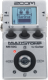# FELIPE

Discussion in 'Zoom MS-50G' started by felipaos, Aug 28, 2023.

1. FELIPEDevice: Zoom Ms-50g
Firmware: 3.10

Name on device: WORSHIP DR
Optimized for: Guitar Amp

Effects chain:SFBXFBSXFBSXF

Effect: "ZNR" (Dynamics / Filter), active - "yes"
"THRSH" = 10
"DETCT" = EfxIn
"Level" = 100

Effect: "Booster" (Overdrive / Distortion), active - "yes"
"Gain" = 82
"Tone" = 50
"Level" = 79

Effect: "MS 1959" (Amp simulator), active - "yes"
"Gain" = 70
"Tube" = 69
"Level" = 108
"Trebl" = 47
"Middl" = 47
"Bass" = 50
"Prese" = 56
"CAB" = OFF
"OUT" = LINE

Effect: "ParaEQ" (Dynamics / Filter), active - "yes"
"Freq1" = 100Hz
"Q1" = 1
"Gain1" = -8
"Freq2" = 6.3kHz
"Q2" = 1
"Gain2" = -7
"Level" = 100

Effect: "AnalogDly" (Delay / Reverb), active - "yes"
"Time" = Quarter
"F.B" = 62
"Mix" = 49
"HiDMP" = 10
"P-P" = MONO
"Level" = 100
"Tail" = On

Effect: "ModReverb" (Delay / Reverb), active - "yes"
"Depth" = 38
"Decay" = 20
"Mix" = 22
"Rate" = 20
"Tone" = 6
"PreD" = 30
"Level" = 72
"Tail" = Off

Note: This is a patch file, you will need to download and install the ToneLib-Zoom software to use the patch.

File size:
440 bytes
Views:
16